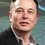# Mirror image equation

Just observed this , I founded it nice so I am sharing it

Now suppose this is the question -

$2x^{8} - 9x^{7} + 20x^{6} - 33x^{5} + 46x^{4} - 66x^{3} + 80x^{2} - 72x + 32 =0$

Let's go in a generalized manner, if we had this equation

$a,b,c...,y>0 , z \in R - \{0\}$

$ax^{2m} + bx^{2m - 1} + cx^{2m -2} + .... + zx^{m} + ...... + cx^2 + bx + a =0$

Keeping $x=0 , ~gives~a=0~so~we~can't~take~x=0$

Now see the beauty,

Dividing the whole equation by $x^m$

$ax^m + bx^{m-1} + cx^{m-2} + ..... + z + ....... + \dfrac{c}{x^{m-2}} + \dfrac{b}{x^{m-1}} + \dfrac{a}{x^m}$

$= a(x^m + \dfrac{1}{x^m}) + b(x^{m-1} + \dfrac{1}{x^{m-1}}) + c(x^{m-2} + \dfrac{1}{x^{m-2}}) + .....+ z =0$

$x + \dfrac{1}{x} = y$

Example(Application) -

$2x^4 + x^3 + x^2 + x + 2 = 0$

See here the coefficients of $x^n$ are a kind of mirror image after $x^2$

Dividing by $x^2$ ( $x \neq 0 ~as~ 2\neq 0$)

$2(x^2 + \dfrac{1}{x^2}) + (x + \dfrac{1}{x}) + 1 =0$

Thus

$2(y^2 - 2) + y + 1 =0$

$2y^2 + y - 3 =0$

$(2y + 3)(y - 1) = 0$

$y = \dfrac{-3}{2} , y =1$

Further we have to substitute y and solve the quadratic

First few

$x^2 + \dfrac{1}{x^2} = y^2 - 2$ (See how lovely this ratio is! $~ (x + \dfrac{1}{x})^2 = x^2 + \dfrac{1}{x^2} + \boxed{2x\times\dfrac{1}{x}}$)

$x^3 + \dfrac{1}{x^3} = y(\boxed{y^2-2}) - \boxed{y} = y^3 - 3y$

$x^4 + \dfrac{1}{x^4} = y(\boxed{ y^3 - 3y}) - \boxed{y^2-2}$

Thus, we can generalize it as -

$x^{n + 1} + \dfrac{1}{x^{n+1}} = \Big(x^n + \dfrac{1}{x^n} \Big)\Big(x + \dfrac{1}{x}\Big) - \Big( x^{n - 1} + \dfrac{1}{x^{n-1}}\Big)$

Writing in terms of t(as most of us write)

$t_{n} = x^n + \dfrac{1}{x^n}$

$\huge{\boxed{t_{n+1} = t_{1}(t_{n}) - t_{n-1}}}$

The question first I asked , solve it yourself - it can be solved by the same methodNote by U Z
4 years, 6 months ago

MarkdownAppears as
*italics* or _italics_ italics
**bold** or __bold__ bold
- bulleted- list
• bulleted
• list
1. numbered2. list
1. numbered
2. list
Note: you must add a full line of space before and after lists for them to show up correctly
paragraph 1paragraph 2

paragraph 1

paragraph 2

[example link](https://brilliant.org)example link
> This is a quote
This is a quote
    # I indented these lines
# 4 spaces, and now they show
# up as a code block.

print "hello world"
# I indented these lines
# 4 spaces, and now they show
# up as a code block.

print "hello world"
MathAppears as
Remember to wrap math in $ ... $ or $ ... $ to ensure proper formatting.
2 \times 3 $2 \times 3$
2^{34} $2^{34}$
a_{i-1} $a_{i-1}$
\frac{2}{3} $\frac{2}{3}$
\sqrt{2} $\sqrt{2}$
\sum_{i=1}^3 $\sum_{i=1}^3$
\sin \theta $\sin \theta$
\boxed{123} $\boxed{123}$

Sort by:

nice...

- 4 years, 6 months ago

FYI, This is called as Reciprocal Equation. Good find! :)

- 4 years, 6 months ago

See how this method is useful in solving this question

- 4 years, 6 months ago

Nice Note , Megh , Thanks For Sharing This ! $\ddot\smile$

- 4 years, 6 months ago

Thanks! I used this trick in a problem in Hall&Knight.

- 4 years, 6 months ago

Yay! Me too :)

- 4 years, 6 months ago

Nice note @megh choksi . I never used this method in general. For quartics, I love this! It would help me a lot!

- 4 years, 6 months ago

Nice note! Did you mean $t_{n+1} = t_{1}t_{n} - t_{n-1}$?

- 4 years, 6 months ago

Yes , thanks for pointing out and reading it

- 4 years, 6 months ago

I just realized that it can be proven easily by $a^{n+1} + b^{n+1} = (a+b)(a^{n}+b^{n}) - ab(a^{n-1}+b^{n-1})$.

- 4 years, 6 months ago

Great , recently I was solving problems of these kinds on brilliant.org , so just I generalized it , nothing more is here

- 4 years, 6 months ago

You're missing an x in the third equation, it should be $cx^{2m-2}$, not just $c^{2m-2}$ unless that was intentional.

- 4 years, 6 months ago

Thanks for pointing out as well as for reading it

- 4 years, 6 months ago